# Find the magnetic field: a. at the center of a 12-turns circular coil of radius 5.00...

Find the magnetic field: a. at the center of a 12-turns circular coil of radius 5.00 cm that carries a current of 4.00 A. (6.03 x 10-4 T) b. at the center of a 600-turns solenoid of length 20.0 cm, radius 1.40 cm that carries a current of 4.00 A (0.015T). c. at the center of a square current loop of side 50 cm that carries a current of 1.5 A (3.4µT). d. due to current-carrying wires of different geometries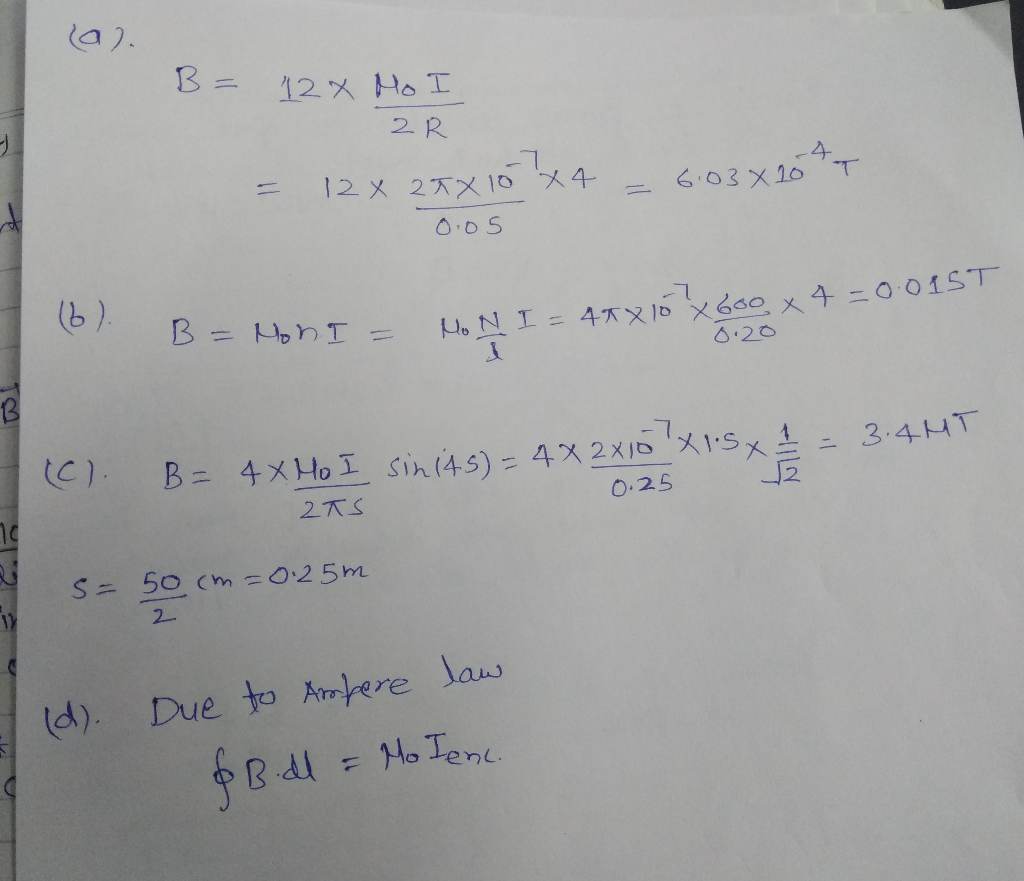#### Earn Coin

Coins can be redeemed for fabulous gifts.

Similar Homework Help Questions
• ### A circular loop of radius 12 cm carries a current of 10 A. A flat coil...

A circular loop of radius 12 cm carries a current of 10 A. A flat coil of radius 0.79 cm, having 59 turns and a current of 1.1 A, is concentric with the loop. The plane of the loop is perpendicular to the plane of the coil. Assume the loop's magnetic field is uniform across the coil. What is the magnitude of (a) the magnetic field produced by the loop at its center and (b) the torque on the coil...

• ### A closely wound circular coil with a diameter of 4.00 cm has 600 turns and carries...

A closely wound circular coil with a diameter of 4.00 cm has 600 turns and carries a current of 0.510 A . What is the magnetic field at the center of the coil?

• ### Questions/Assignments an expression for the magnetic field at the center of circular loop of current carrying...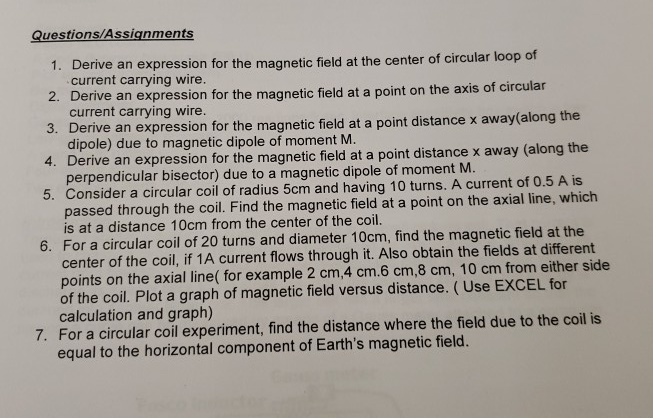Questions/Assignments an expression for the magnetic field at the center of circular loop of current carrying wire erive an expression for the magnetic field at a point on the axis of circular current carrying wire. 3. D erive an expression for the magnetic field at a point distance x away(along the dipole) due to magnetic dipole of moment M. 4. Derive an expression for the magnetic field at a point distance x away (along the perpendicular bisector) due to a...

• ### A square conducting loop with side length a = 1.25 cm is placed at the center...

A square conducting loop with side length a = 1.25 cm is placed at the center of a solenoid 40.0 cm long with a current of 4.60 A flowing through its 470 turns, and it is aligned so that the plane of the loop is perpendicular to the long axis of the solenoid. The radius of the solenoid is 5.00 cm. (a) What is the magnetic flux through the loop? Wb (b) What is the magnitude of the average emf...

• ### Please answer clearly Problem 3 a) Find the magnetic field H at the center of a...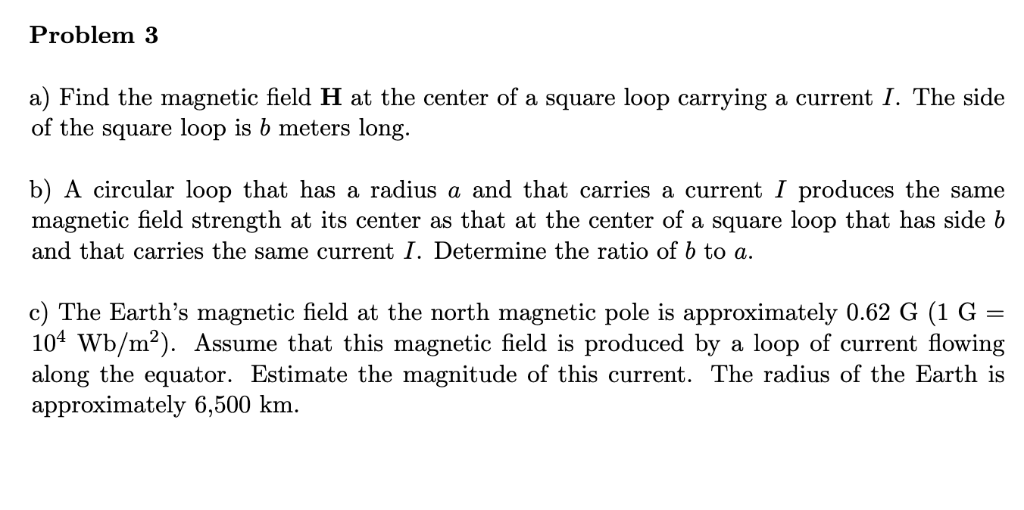Please answer clearly Problem 3 a) Find the magnetic field H at the center of a square loop carrying a current I. The side of the square loop is b meters long. b) A circular loop that has a radius a and that carries a current I produces the same magnetic field strength at its center as that at the center of a square loop that has side b and that carries the same current I. Determine the ratio of...

• ### A circular coil of 200 turns, with a radius of 8 cm, is dropped vertically into...

A circular coil of 200 turns, with a radius of 8 cm, is dropped vertically into a very long solenoid, which has a radius of 12 cm, 300 turns / m, and which conducts a current of 3 A. What is the value of the emf induced in the coil that is in free fall, due to the magnetic field of the solenoid?

• ### 1. A coil is located at the center of a long solenoid of radius R- 15...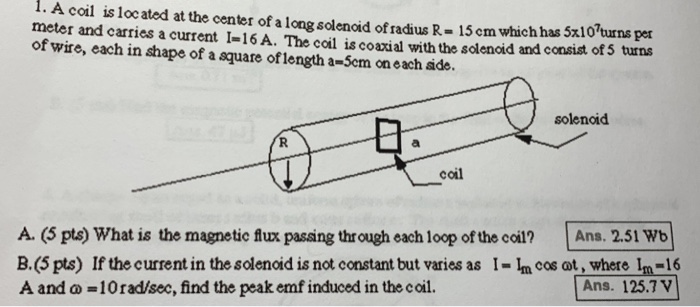1. A coil is located at the center of a long solenoid of radius R- 15 cm which has 5x10'turns per meter and carries a current I-16A. The coil is coaxial with the solenoid and consist ors of wire, each in shape of a square oflength a-5cm on each ade. solenoid coil A (5 pts) What is the magnetic fux passing through each loop of the coil? Ans. 2.51 Wb B.(5 pts) If the current in the solenoid is not...

• ### A circular coil of 200 turns, with a radius of 8 cm, is dropped vertically into...

A circular coil of 200 turns, with a radius of 8 cm, is dropped vertically into a very long solenoid, which has a radius of 12 cm, 300 turns / m, and which conducts a current of 3 A. What is the value of the emf induced in the coil that is in free fall, due to the magnetic field of the solenoid ?. Express your answer in detail.

• ### Find the magnetic field strength inside the coil at its midpoint/ the magnetic flux through a...

A 420 turn solenoid of length 39.0 cm and radius 3.30 cm carries a current of 5.20 A. Find the following.(a) the magnetic field strength inside the coil at its midpoint(b) the magnetic flux through a circular cross-sectional area of the solenoid at its midpoint

• ### 1. The armature of an ac generator is a circular coil with 292 turns and radius...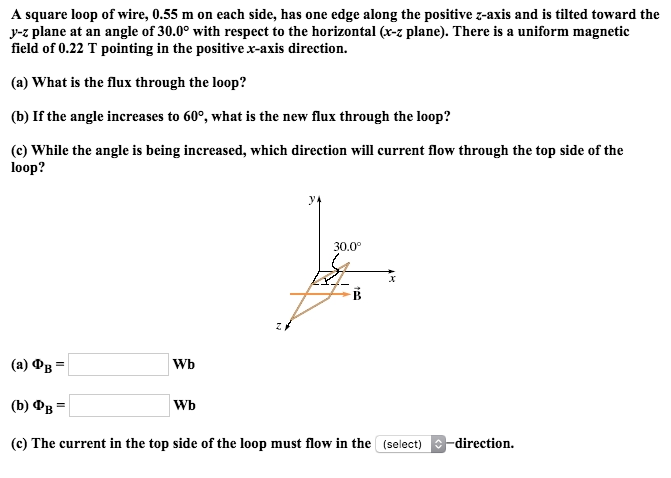1. The armature of an ac generator is a circular coil with 292 turns and radius 4.6 cm. When the armature rotates at 939 rpm, the amplitude of the emf in the coil is 21.3 V. What is the strength of the magnetic field (assumed to be uniform)? 2. The armature of an ac generator is a rectangular coil 27.0 cm by 17.9 cm with 294 turns. It is immersed in a uniform magnetic field of magnitude 3.0 T. If...